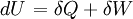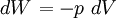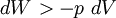# First law of thermodynamics

The first law of thermodynamics is a statement of the conservation of energy for thermal processes:$\left.dU\right.=\delta Q+ \delta W$

Where U is the internal energy of the system, i.e. ignoring the external energy of the system, for example motion of the center of mass of the system, or the presence of an external field. Q is the net heat entering the system and W is the work done upon the system.

For reversible changes$\left.dW\right.=-p~dV$

and for irreversible changes$\left.dW\right. > -p~dV$

where$p$ is the pressure and$V$ is the volume.

For an adiabatic system (i.e. a system in which no heat enters or leaves) then if two bodies of different temperatures are placed in contact and then separated then the sum of the heat within the system is unchanged (see adiabatic process. This is conservation of energy. The first law of thermodynamics does not provide information on the direction of the heat transfer (if any). In other words, the common experience that the hotter object gives heat to the colder object, the direction of heat flow from hot to cold, is left to the second law of thermodynamics.